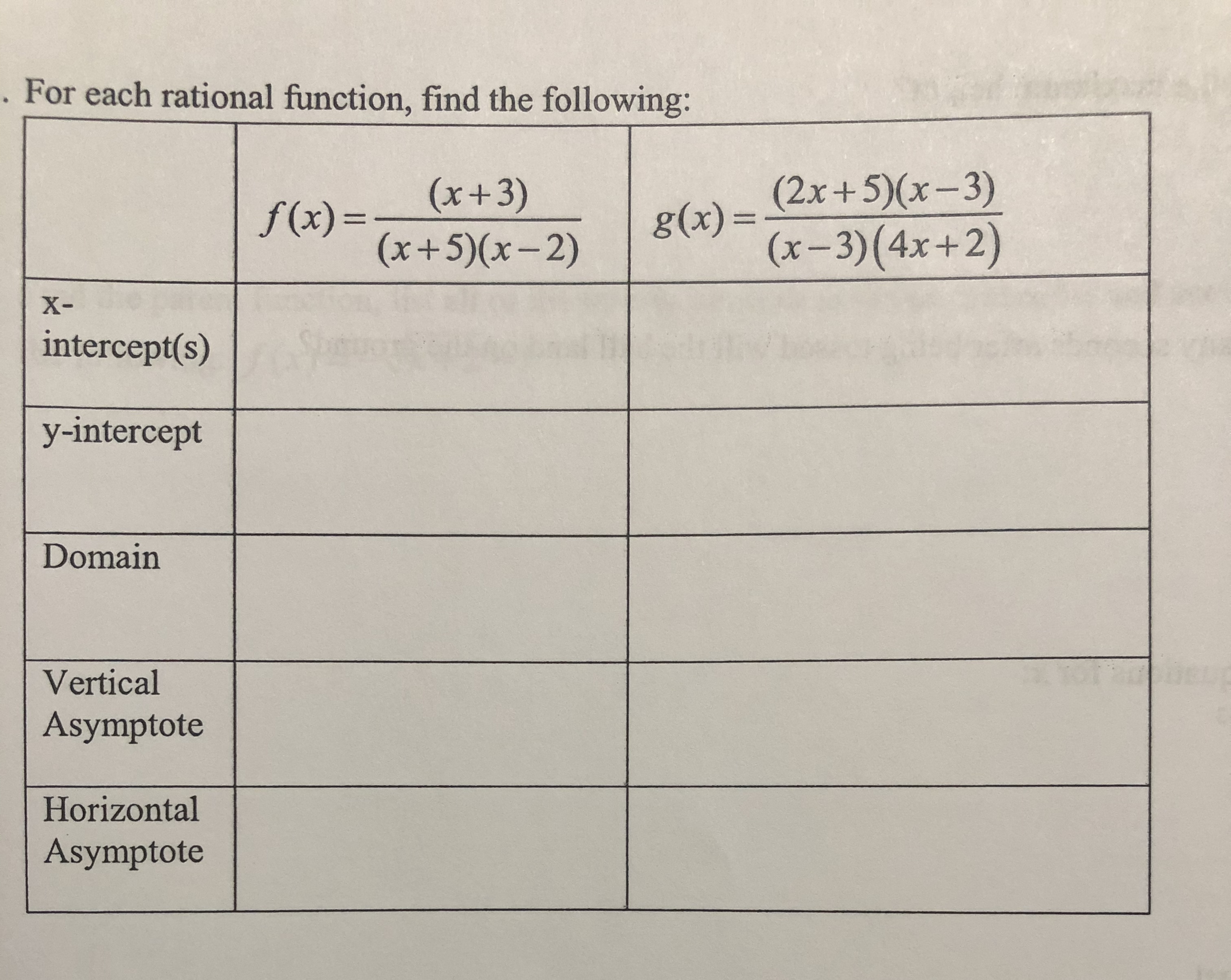# . For each rational function, find the following:(2x+5)(x-3)g(x)3=(x-3)(4x+2)(x+3)(x+5)(x-2)f(x) =%3DX-intercept(s)y-interceptDomainVerticalAsymptoteHorizontalAsymptote

Question
28 viewshelp_outlineImage Transcriptionclose. For each rational function, find the following: (2x+5)(x-3) g(x)3= (x-3)(4x+2) (x+3) (x+5)(x-2) f(x) = %3D X- intercept(s) y-intercept Domain Vertical Asymptote Horizontal Asymptote fullscreen
check_circle

Step 1

Note: We are authorized to answer three sub-parts at a time. Since you have asked more than three sub-parts at time, we are answering the first three only. Please, re-post your question separately for the remaining subparts.

Step 2

The given functions are

Step 3

To find:

• X-intercepts of f(x) and g(x).
• Y-intercepts of f(x) and ...

### Want to see the full answer?

See Solution

#### Want to see this answer and more?

Solutions are written by subject experts who are available 24/7. Questions are typically answered within 1 hour.*

See Solution
*Response times may vary by subject and question.
Tagged in

### Algebra